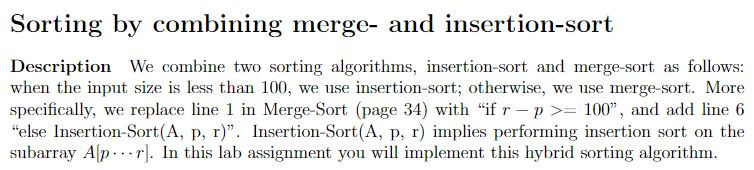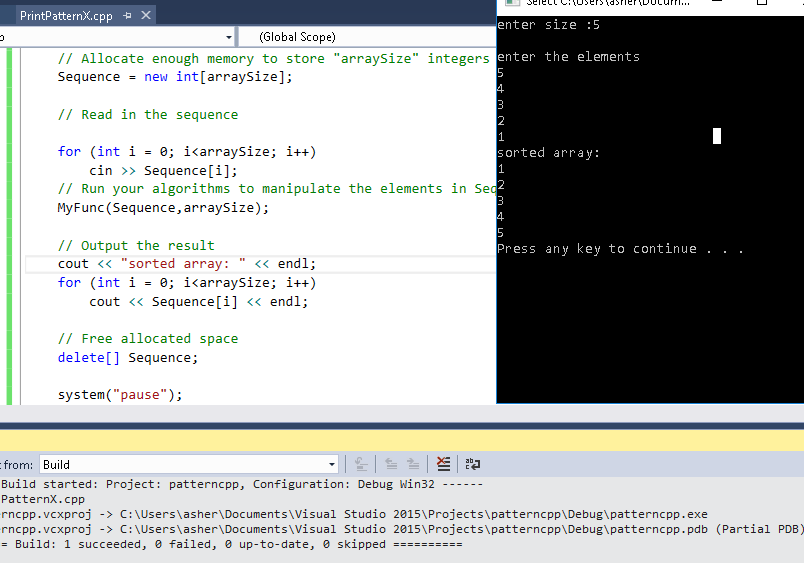# Answered Essay: Sorting An array with c++

Sorting An array with c++Follow the codes format below! thanks so much if you can help me with this!!!!!!!!!

#include <iostream>
#include <stdlib.h>
#include <string>

using namespace std;

void MyFunc ( int *array ) {
// …
}

int main(int argc,char **argv) {

int *Sequence;
int arraySize;

// Get the size of the sequence
cin >> arraySize;

// Allocate enough memory to store “arraySize” integers
Sequence = new int[arraySize];

// Read in the sequence
for ( int i=0; i<arraySize; i++ )
cin >> Sequence[i];

// Run your algorithms to manipulate the elements in Sequence
MyFunc(Sequence);

// Output the result
for(int i=0; i<arraySize; i++)
cout << Sequence[i] << endl;

// Free allocated space
delete[] Sequence;
}

Show transcribed image textSorting by combining merge- and insertion-sort Description We combine two sorting algorithms, insertion-sort and merge-sort as follows: when the input size is less than 100, we use insertion-sort; otherwise, we use merge-sort. More specifically, we replace line 1 in Merge-Sort (page 34) with “If r-p >= 100”, and add line 6 else Insertion-Sort(A, p, r). Insertion-Sort(A, p, r) implies performing insertion sort on the subarray Alpr. In this lab assignment you will implement this hybrid sorting algorithm.

#include <iostream>

#include <stdlib.h>

#include <string>

using namespace std;

void insertionSort(int a[], int p, int r)

{

int i, key, j;

for (i = p; i < r; i++)

{

key = a[i];

j = i – 1;

while (j >= p && a[j] > key)

{

a[j + 1] = a[j];

j = j – 1;

}

a[j + 1] = key;

}

}

void merge(int a[], int q, int p, int r)

{

int i, j, k;

int m1 = q – p + 1;

int m2 = r – p;

int *L;

int *R;

L = new int[m1];

R = new int[m2];

/* Copy data to temp arrays L[] and R[] */

for (i = 0; i < m1; i++)

L[i] = a[q + i];

for (j = 0; j < m2; j++)

R[j] = a[p + 1 + j];

i = 0; // Initial index of first subarray

j = 0; // Initial index of second subarray

k = q; // Initial index of merged subarray

while (i < m1 && j < m2)

{

if (L[i] <= R[j])

{

a[k] = L[i];

i++;

}

else

{

a[k] = R[j];

j++;

}

k++;

}

while (i < m1)

{

a[k] = L[i];

i++;

k++;

}

while (j < m2)

{

a[k] = R[j];

j++;

k++;

}

delete[] L;

delete[] R;

}

void mergeSort(int a[], int p, int r)

{

if (r – p>=100)

{

int q = (p + r) / 2;

mergeSort(a, p, q);

mergeSort(a, q +1, r);

merge(a, p, q, r);

}

else

{

insertionSort(a, p, r);

}

}

void MyFunc(int *array,int size) {

mergeSort(array, 0, size);

}

int main(int argc, char **argv) {

int *Sequence;

int arraySize;

// Get the size of the sequence

cout << “enter size :”;

cin >> arraySize;

cout << “nenter the elements ” << endl;

// Allocate enough memory to store “arraySize” integers

Sequence = new int[arraySize];

// Read in the sequence

for (int i = 0; i<arraySize; i++)

cin >> Sequence[i];

// Run your algorithms to manipulate the elements in Sequence

MyFunc(Sequence,arraySize);

// Output the result

cout << “sorted array: ” << endl;

for (int i = 0; i<arraySize; i++)

cout << Sequence[i] << endl;

// Free allocated space

delete[] Sequence;

system(“pause”);

}

—————————————–Calculate your paper price
Pages (550 words)
Approximate price: -

Help Me Write My Essay - Reasons:Best Online Essay Writing Service

We strive to give our customers the best online essay writing experience. We Make sure essays are submitted on time and all the instructions are followed.Our Writers are Experienced and Professional

Our essay writing service is founded on professional writers who are on stand by to help you any time.Free Revision Fo all Essays

Sometimes you may require our writers to add on a point to make your essay as customised as possible, we will give you unlimited times to do this. And we will do it for free.Timely Essay(s)

We understand the frustrations that comes with late essays and our writers are extra careful to not violate this term. Our support team is always engauging our writers to help you have your essay ahead of time.Customised Essays &100% Confidential

Our Online writing Service has zero torelance for plagiarised papers. We have plagiarism checking tool that generate plagiarism reports just to make sure you are satisfied.Our agents are ready to help you around the clock. Please feel free to reach out and enquire about anything.

Try it now!

## Calculate the price of your order

Total price:
\$0.00

How it works?

Follow these simple steps to get your paper doneFill in the order form and provide all details of your assignment.Proceed with the payment

Choose the payment system that suits you most.Receive the final file

Once your paper is ready, we will email it to you.

HOW OUR ONLINE ESSAY WRITING SERVICE WORKS

Let us write that nagging essay.## Submit Your Essay/Homework Instructions

By clicking on the "PLACE ORDER" button, tell us your requires. Be precise for an accurate customised essay. You may also upload any reading materials where applicable.Pick A & Writer

Our ordering form will provide you with a list of writers and their feedbacks. At step 2, its time select a writer. Our online agents are on stand by to help you just in case.Editing (OUR PART)

At this stage, our editor will go through your essay and make sure your writer did meet all the instructions.